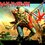# Introduction to recurrence relations

This post is a guide to the art of breaking a complex problem into simpler versions of the same problem namely the art of problem solving by recurrence relations.it is a part of this setimg

In many problems related to combinatorial counting there involves an integer parameter $n$.This $n$ denotes either some set or multiset in the problem or size of combinations etc.Thus a counting problem is often not just one problem but a sequence of individual problems.There are some algebraic methods for solving counting problems involving an integer parameter $n$ which most of the time leads to an explicit formula.

For obtaining a recurrence relation,we must proceed along the following steps.

$\textbf{Obtain a base case}$

At first we must solve a simpler version of the problem at hand.We will later use this result obtained to solve the larger problem.So example in the Fibonacci sequence we have the first two terms as base cases.That is $F_1 = 0$ and $F_2 = 1$.

$\textbf{Formulate a recursive definition:}$

Next we must break the larger problem down into smaller version of the same problem and continue this process until we arrive at a base case.Then we have to use this base case either to obtain a generic term or an explicit formula if possible.

As an example for the Fibonacci sequence we have the recursive definition $F_n = F_{n-1} + F_{n-2}$.So using this definition and the base cases we can find any term of the sequence that we want.7 years, 2 months ago

This discussion board is a place to discuss our Daily Challenges and the math and science related to those challenges. Explanations are more than just a solution — they should explain the steps and thinking strategies that you used to obtain the solution. Comments should further the discussion of math and science.

When posting on Brilliant:

• Use the emojis to react to an explanation, whether you're congratulating a job well done , or just really confused .
• Ask specific questions about the challenge or the steps in somebody's explanation. Well-posed questions can add a lot to the discussion, but posting "I don't understand!" doesn't help anyone.
• Try to contribute something new to the discussion, whether it is an extension, generalization or other idea related to the challenge.

MarkdownAppears as
*italics* or _italics_ italics
**bold** or __bold__ bold
- bulleted- list
• bulleted
• list
1. numbered2. list
1. numbered
2. list
Note: you must add a full line of space before and after lists for them to show up correctly
paragraph 1paragraph 2

paragraph 1

paragraph 2

[example link](https://brilliant.org)example link
> This is a quote
This is a quote
    # I indented these lines
# 4 spaces, and now they show
# up as a code block.

print "hello world"
# I indented these lines
# 4 spaces, and now they show
# up as a code block.

print "hello world"
MathAppears as
Remember to wrap math in $$ ... $$ or $ ... $ to ensure proper formatting.
2 \times 3 $2 \times 3$
2^{34} $2^{34}$
a_{i-1} $a_{i-1}$
\frac{2}{3} $\frac{2}{3}$
\sqrt{2} $\sqrt{2}$
\sum_{i=1}^3 $\sum_{i=1}^3$
\sin \theta $\sin \theta$
\boxed{123} $\boxed{123}$